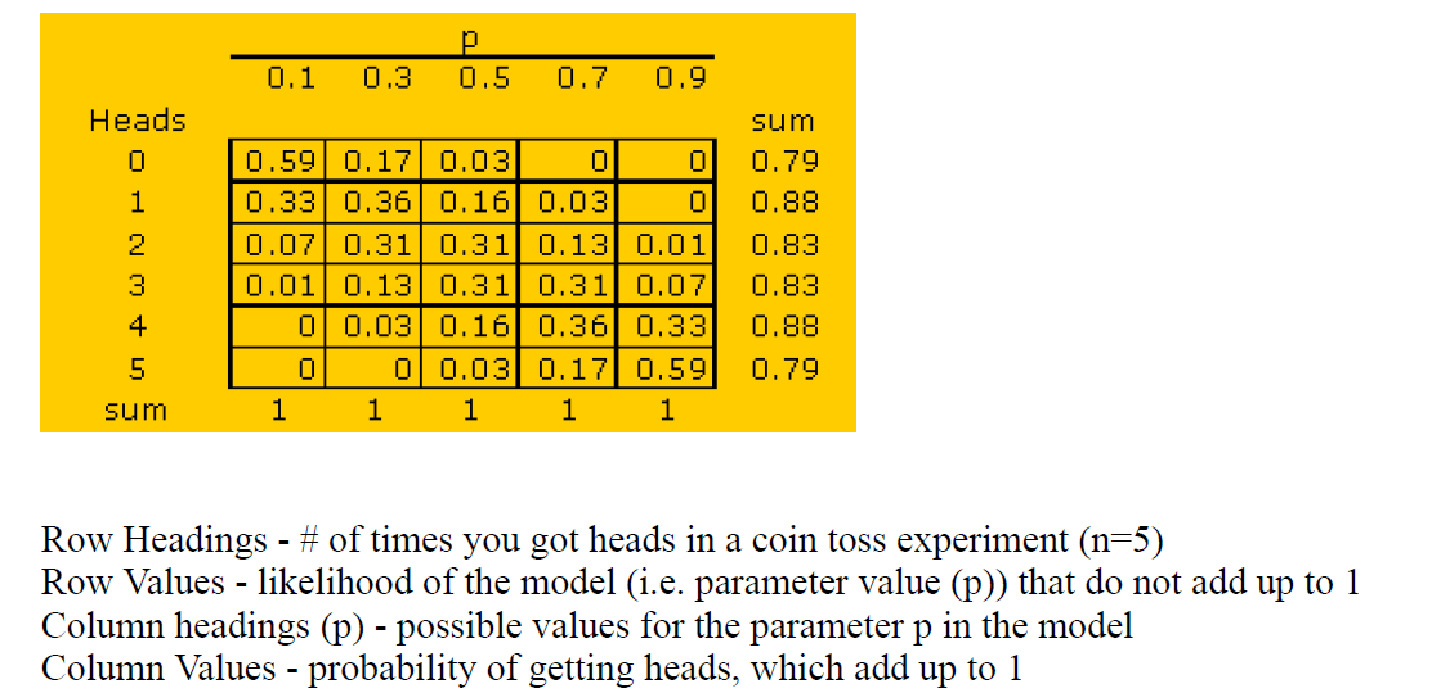# Likelihood.vs.Probablity

1. Reference

LikelyhoodFunction_The world is a complex place.pdf1. ExampleLikelihood:  when an event happened (for example, Heads=3), what is the probability of each possible model parameter.

Probability: If I know the model parameter (for example p=0.5), what is the probability for some events?

So, values in the context of one row are called likelihood.

Values in the context of one Column are called probability.

Just similar as Chinese saying, “横看成岭侧成峰”. You can interpret data in different context.